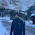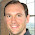## Monday, June 27, 2016

### Salesforce Wave Explained - Cogroup

Lately, I have received a few questions around ways to combine data in Salesforce Wave.  Previous posts have covered combining internal and external data with data flow transformations.  If combining data while loading is not an option, there are other ways to get the results you need.  While the group statement is a staple of SAQL, its sibling cogroup has a staring role when combining multiple input streams.  Let's take a closer look at cogroup and how we use it to combine two datasets.

## Dataset Setup

To start, we need multiple datasets to work with.  Fort the first data set, start with the Sales data from my sales data generator.  As a refresher, this is a simple data set of Account, Product, Period and Price.  You can use the sales data generator to create sample data, or use the sample file.  Now for our second data set.  I have added a Quota.csv file to the accompany the sales data.  This data file has three columns - Product, Period and Amount.

The first task is to import each file as its own data set - Sales and Quota respectively.  Keep in mind to set your date format when loading the csv - Month/Day/Year.  I have sample data set json in the analytics-cloud-cogroup github project.  Now, let's build some dashboards.

## Sales & Quota Lenses

First, create a simple lens of Sales data, grouped by the Period Year-Month and the sum of Price and clip to a dashboard.

Next, do the same for Quota data with the sum of Amount.

Now, we can add these charts to a dashboard.  While the dashboard is interesting, it's not a particularly useful example.  It would me much better to see quota against our sales performance.  The next step is diving into SAQL to make it happen.

## Diving Into SAQL

From the dashboard, switch into the dashboard JSON (CMD-E on Mac, CTRL-E on Windows).  First, we will work on creating SAQL statements for each step.  Let's start with the Quota step.  I have renamed the step to Quota from the default name for ease of identification.  If you do this after creating a widget, be sure to update the Step reference in the corresponding widget.

Here is the basic SAQL statement that we will add.  First, load the Quota data set, next group by the Period Year and Month, then generate the sum of Amount for each.

`"pigql": "q = load \"Quota\"; `
`q = group q by (Period_Year, Period_Month); `
`q = foreach q generate Period_Year + \"~~~\" + Period_Month as `
`'Period_Year~~~Period_Month', sum(Amount) as sum_Amount;"`

The Sales SAQL statement is very similar - different dataset a field names.

`"pigql" : "q = load \"Sales\"; `
`q = group q by (Period_Year, Period_Month); `
`q = foreach q generate Period_Year + \"~~~\" + Period_Month as `
```'Period_Year~~~Period_Month', sum(Price) as sum_Price"
```

A quick switch back to the run-time verifies that the statements have been properly added and the dashboard still works.

## Introducing Cogroup

The next task is to add a new step to the dashboard JSON that will hold Quota with Sales.  Start by copying the Quota step, pasting it into the steps section in the dashboard JSON and renaming to Quota_with_Sales.

`"pigql": "q = load \"Quota\"; r = load \"Sales\";`

Then, we can change the group statement into cogroup.  Change the assignment to s instead of q.  Then include r in the statement as well.  We will need each input stream in the cogroup as a separate variable, as we will need to reference the stream in the foreach statement.

`s = cogroup q by (Period_Year, Period_Month), `
`r by (Period_Year, Period_Month);`

There are a few adjustments to the foreach statement.  After changing to s, we also need to include either the q or r stream as the qualifier for each dimension or measure.  For example, adding q to the Period Year, Period Month and Amount and r to the Price.

`s = foreach s generate q.Period_Year + \"~~~\" + q.Period_Month as `
`'Period_Year~~~Period_Month', `
`sum(q.Amount) as sum_Amount, `
`sum(r.Price) as sum_Price;"`

The last step before switching back to the run time is adding the Price measure to the Measures section.  Add it before sum_Amount

```"measures" : [
[
"sum",
"Price"
],
[
"sum",
"Amount"
]
]
```

Now switch back to the run time, add a combo chart with Quota_with_Sales as its source.  We have a more great visualization to use.  A chart that shows the Quota (red line) with bars that show how actual Sales performance has measured up.

So what is going on behind the scenes with our new visualization and the cogroup statement?  By cogrouping both Quota and Sales together, we can match up each data set on Year and Month and see results where there are matches.  Think of cogroup being similar to a join when working with SQL.  For January 2014 we pull the quota value along with the actual sales.

At this point, an observant reader may point out that I had Quota data for multiple months, but the combined chart only shows where Sales data matches.  Is there a way to see the Quota values even when there is not a matching Sales year and month?   For example, looking at Quota for the coming quarter?

Yes, there is.  Just like with SQL there are right, left and full cogroups.  With right or left, all of the values one dataset are returned along with the matches from the other.  Similarly, a full cogroup takes all the values from both data sets and lines up the matches.

Let's head back to design mode and update our SAQL statement. Pulling all the Quotas is a simple change of adding "left" to our cogroup statement.

`s = cogroup q by (Period_Year, Period_Month) left, `
`r by (Period_Year, Period_Month); `

Now, we can see Sales versus plan and our expected performance moving forward.

## Final Thoughts

Cogroup is a great way to combine data on a dashboard.  There are a few additional considerations to keep in mind.  When using a full outer cogroup, you may not have values in one of the datasets.  In my example, this could mean the Year and Month would be returned as null values if I tried to pull them from the Sales dataset (r).

To handle this case, use the coalesce function.  Coalesce will return the first non-null value of the options provided to it.  For example, "q = foreach q generate coalesce(q.Period_Year, r.Period_Year);" will first return the value from q (Quota) and then r (Sales) if quota is blank.

Finally, cogroup is not limited to two datasets.  You can use cogroup to combine multiple sets of data.  You can even perform self cogroups using the same dataset.  Just be sure to load the dataset twice into different input streams.

Now with the power of cogroup at your disposal you can create some pretty cool dashboards that help met business needs.  For reference, the dataset and dashboard examples used in this tutorial are available in my github repository.

1.Thanks for posting this. its well explained !!!!

2.Hi I have doubt in full outer join.Can we use the same datasets with didfferent filter criteria

3.Yes, you can use the same data set in a cogroup. However, you must load it multiple times

"pigql": "q = load \"Quota\"; r = load \"Quota\"; s = cogroup q by (Period_Year, Period_Month),
r by (Period_Year, Period_Month);

It would not work if you loaded one data set and attempted to use it twice.

4.Hi Carl. Great post. Is there any way to get fields that are not in both data sets? For example, could you cogroup these two sets, but still pull in the Product Field from the Quota data set even if it wasn't in the Sales data set?

5.Yes, you can use a left (or right) cogroup just like with a SQL statement. The key is to put the keyword left after the first dataset and before the comma and the second dataset.

6.Hi Carl. I think I was probably not clear in my explanation. I want to do something like this:
s = cogroup q by (fieldA, fieldB) left, r by (fieldA, fieldB);
s = foreach s generate fieldA, fieldB, CountFieldFromR

What I'm trying to do is get an overlay of two data sets for a HeatMap. In this case, fieldA would represent rows that the two sets have in common, fieldB would represent the columns that the two have in common, and the CountFieldFromR is a value from set r that would be the value in the Heatmap. Basically, q is a "template" of targets of the intersections of fieldA and fieldB we are interested in. If there is no row for a fieldA/FieldB combination in set q -- even if it is present in set r -- the combo comes out NULL, and doesn't show up in the heatmap (shows as white). But I can't seem to get the values for CountFieldFromR into the foreach statement for values for the Heat in the heatmap.

7.Are you having trouble with the syntax for the saql statement, which should be similar to
s = foreach s generate q.fieldA, q.fieldB, count(r)

Or are you encountering an issue with the returned data? If it is with the returned data, try using a coalesce(CountFieldFromR, 0) in the saql to change any nulls to 0 (or the value that makes sense in your case.

8.Hello Carl, Thank you for the wonderfull explanation on Co group and i am working on a similar requirement using compare table and get the percentage growth by Account, by comparing 2018 and 2019 sales data and following is the code:

q_A = filter q by date('Date__c_Year', 'Date__c_Month', 'Date__c_Day') in ["current year".."current year"];

q_B = filter q by date('Date__c_Year', 'Date__c_Month', 'Date__c_Day') in ["1 year ago".."1 year ago"];

result = cogroup q_A by ('Parent_Account_Name__c') full, q_B by('Parent_Account_Name__c');

result = foreach result generate coalesce(q_A.'Parent_Account_Name__c', q_B.'Parent_Account_Name__c') as 'Parent_Account_Name__c', coalesce(sum(q_A.'Sales__c'),0) as 'A',coalesce(sum(q_B.'Sales__c'),0) as 'B', 'C" ;

result = order result by ('Parent_Account_Name__c' asc);
result = limit result 2000;

In this i am trying to achieve column C with the 2019 sales -2018 sales/2019 sales

Any help is greatly appreciated

9.For this, I would use a compare table. Column A is Sum of Sales filtered to 2019. Column B is Sum of Sales filtered to 2019. Then use a formula for column C. It would be (B-A)/B.

You may also want to use a coalesce(A, 0) or coalesce(B, 0) if there will be Accounts without sales for one of the years.